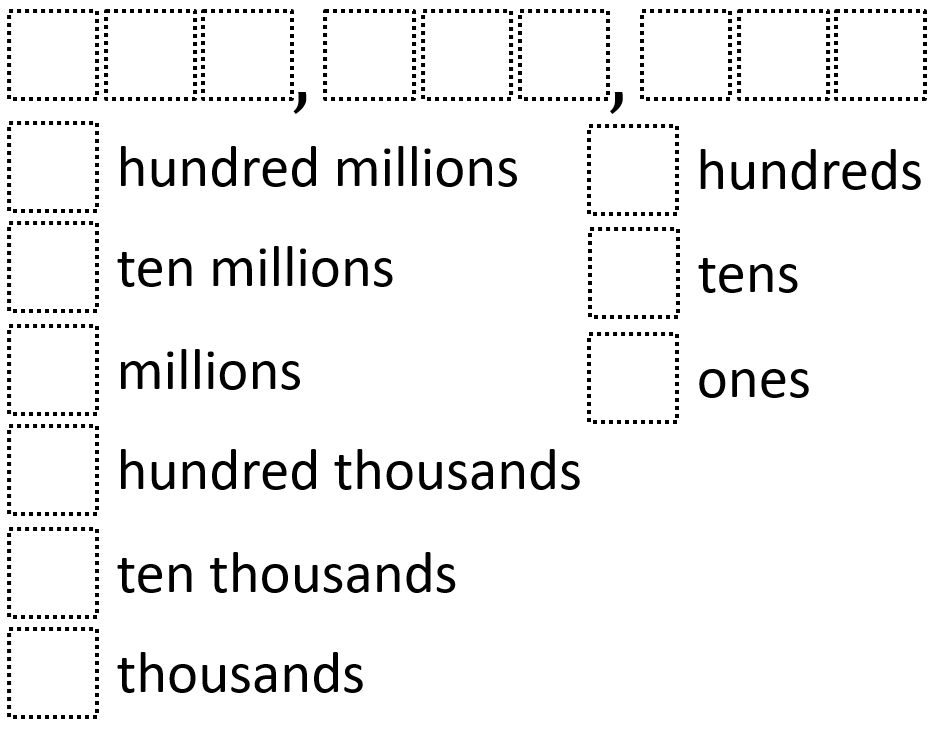# Place Value

Directions: Use the digits 1 to 9, at most TWO times each, to create a nine-digit number and its corresponding place values.### Hint

How do we know what each digit in the nine-digit number represents?

There are many answers. The nine-digit number should use the same digits as the corresponding place values.

Source: Owen Kaplinsky

## Add Fractions with Decimal Sums

Directions: Using the digits 1 to 9 at most one time each, place a digit …

1.Please could you help me understand the place value question of September 7,2018.

•You need to use the digits 1-9 to make a nine digit number, and then use the same digits and organize them into their place values.

2.Chelsea McClellan

Wondering how this is DOK 2? I might be missing something? Is it possible to scramble the corresponding place values so they are not in order? Perhaps ask students to create the largest or smallest 9-digit number. We also found the directions a bit confusing. New to open middle folks interpret them as allowing 2 of the same digit in the 9-digit number. As a long-time user of open middle, I fully understood the intent of the directions.

3.I don’t understand the directions either. Could you give an example?

4.I had the students make 3 different numbers with different rules associated.

1.) I did this as instructed. Use the digits 1-9 at most TWO times each to create a 9 digit number and it’s corresponding place values. We discussed our numbers with a peer.

2.) I had the students create a second number that is larger or smaller than the first. Use the digits 1-9 at most TWO times each to create a 9 digit number and it’s corresponding place values that is LARGER or SMALLER than your first number.

3.) Finally, I had the students create numbers that are in the middle of the two numbers that they created. Use the digits 1-9 at most TWO times each to create as many 9 digit numbers as you can that fall in the middle of your two previous numbers. Prove why you know they are in the middle.

This was used for a Grade 5 class.

•Awesome way to challenge thinking Jordan, thanks for sharing.